# Lesson Notes By Weeks and Term - Primary 2

Multiplication using arrays

TERM: 3rd Term

WEEK: 3

CLASS: Primary 2

AGE: 7 years

DURATION: 5 periods of 40 minutes each

DATE:

SUBJECT: Mathematics

TOPIC: Multiplication using arrays

SPECIFIC OBJECTIVES: At the end of the lesson, the pupils should be able to

1. Solve multiplication number sentences.
2. Create and solve multiplication number sentences using array diagrams.
3. Solve multiplication word problems using a multiplication table.

INSTRUCTIONAL TECHNIQUES: Explanation, question and answer, demonstration, practical

INSTRUCTIONAL MATERIALS: Array diagram, enlarged array diagram, 1 to 5 enlarged multiplication table, multiplication cards (×2, ×3, ×4 and ×5), scrap paper (2 sheets per learner)

PERIOD 1: Multiplication using arrays

PRESENTATION

TEACHER’S ACTIVITY

PUPIL’S ACTIVITY

STEP 1

MENTAL MATHS

The teacher begins the lesson with some mental calculations

Calculate

1 4 × 3

2 3 × 4

3 2 × 4

4 4 × 2

5 3 × 5

6 5 × 3

7 4 × 5

8 5 × 4

9 2 × 5

10 5 × 2

Pupils respond and participate

STEP 2

CONCEPT

DEVELOPMENT

The teacher

• Gives each learner an array diagram.

• Puts an enlarged array diagram on the board.

• Writes the following problem on the board (you will use this problem for

Activity 1 and 2):

You have 3 boxes which each have 4 oranges in them.

How many oranges do you have?

• Reads the problem several times.

• Lets the learners read the problem until they read it fluently.

• Underlines the numbers 3 and 4.

• Underlines the question (How many oranges do you have?) with wavy line.

• Lets the learners represent the story with bottle tops.

• Lets them write a multiplication number sentence for the problem in their classwork books.

• Writes the multiplication number sentence on the board for correction: 3 × 4 = __

• Says: Let’s find the answer using the array diagram.

• Gives learners time to use the array diagram to solve the problem. This is experimental.

You will show them how to use the array diagram after they have had a bit of time to experiment.

• Asks: How did you use the array diagram to find the answer?

• Lets some learners explain to the class how they found the answer to the problem.

• Shows learners how to use the array diagram step by step, so that learners can follow what you do on the board. The right way of using the array diagram to show 3 × 4 = __ is

as follows.

• Hides away some of the columns by holding paper (1) so that you leave 4 columns open.

The number of columns gives us the size of the group: 3 × 4 = __ needs groups of 4 (see below).

• Then, hides away some of the rows, using a second piece of paper (2) as shown below.

The number of rows gives us the multiple we need (in 3 × 4 = __ the multiple is 3 x)

Paper 2 can be moved up and down, to show different multiples of 4.

The diagram below shows an array and papers to show 3 × 4 = 12. (NOTE: It is not 4 × 3. This would be shown by 4 rows with 3 dots in each row.)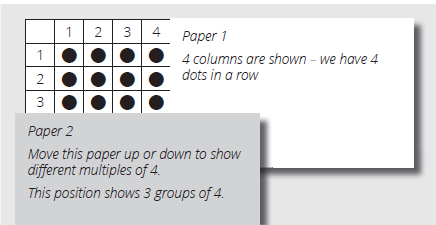By sliding paper 2 down from row 1 to row 3, row by row, learners can count the first

three multiples of 4: 4, 8 and 12. (and so on)

Lets the learners record the number sentence and its answer in their classwork books. (3 × 4 = 12, 12 oranges)

Repeats the above steps using the array to show 4 × 3 = __

Lets the learners use the array diagram to show the multiple and find the answer.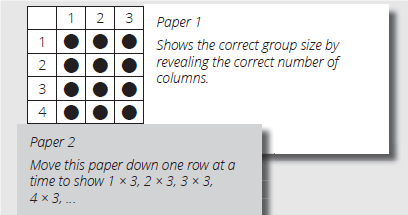Helps learners to see that they can fi nd the answers by counting in 3s.

As the number of groups (represented by the rows) increases, so the answer increases by 3 each time.

The array diagram should always be used in the way shown above, i.e. by hiding unnecessary parts with paper.

Discusses with the learners the relationship between 3 × 4 and 4 × 3 (The answer is the

same. It is 12).

CLASS ACTIVITY

The teacher

Changes the numbers in the word problem as follows:

You have 4 boxes which each have 5 oranges in them.

How many oranges do you have?

Underlines the numbers 4 and 5.

Underlines the question (How many oranges do you have?) with wavy line.

Shows the story with tapes or drawing on the board.

Lets the learners represent the story with bottle tops.

Lets them write the multiplication number sentence in their classwork books.

Writes the following multiplication number sentence on the board for correction.

4 × 5 = __

Reminds learners how to use the array diagram to help them solve the problem.

Lets a learner explain to the class how she/he solved the problem on the array diagram.

Encourages learners to verbalise that the number 4 tells us the number of rows while the number 5 tells us the number of columns.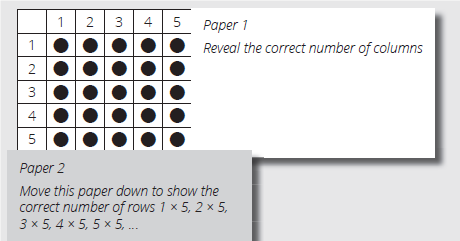• Asks one of the learners (from each pair) to move the papers so that they now show the product 4 × 5. (They should show 4 rows with 5 dots in each row.)

• Lets the learners record the number sentence and its answer in their classwork books.

(4 × 5 = 20, 20 oranges)

Pupils pay attention and participate

STEP 3

CLASS-WORK

NOTE: The learners should use their array diagrams to calculate the answers to

the problems.

1 Mangoes are N5 each. Mercy buys 3 mangoes. How much does she pay?

a Number sentence: __________________________ .

b She pays N _______ .

2 I have 4 packets of sweets. There are 2 sweets in each packet. How many sweets

do I have?

a Number sentence: ______________________________ .

b You have _____  sweets.

Pupils attempt their class work

STEP 4

HOME-WORK

Complete the table

 Reverse the table 1 4 x 3 = 12 2 5 x 2 = 10 3 3 x 2 = 6 4 4 x 1 = 4 5 3 x 5 = 15

The pupils writes it in their homework book

STEP 5

SUMMARY

The teacher summarizes by reminding the pupils that in this lesson we have learnt to solve multiplication number sentences using array diagrams.

She marks their class works, makes corrections where necessary and commends them positively

PERIOD 2: Creating multiplication stories

PRESENTATION

TEACHER’S ACTIVITY

PUPIL’S ACTIVITY

STEP 1

MENTAL MATHS

The teacher begins the lesson with some mental calculations

What is

1 5 × 4

2 4 × 2

3 2 × 3

4 3 × 3

5 5 × 2

6 2 × 2

7 5 × 5

8 4 × 4

9 5 × 3

10 4 × 3

Pupils respond and participate

STEP 2

CONCEPT

DEVELOPMENT

The teacher

• Gives each learner an array diagram.

• Writes 2 × 5 = __ on the board.

• Puts an enlarged array diagram on the board.

• Says: Let’s make up a multiplication word problem for 2 × 5.

• Gives learners time to think.

• Lets them tell their word problems to the class.

• Writes a few of the word problems on the board.

• Checks that all of the problems are talking about 2 groups of 5 or 5 groups of 2.

(Problems must be a story about 2 groups 5.)

• Selects one problem and ask learners to use the array diagram to solve the problem.

• Asks a learner to show how to find the answer using the array diagram on the board.

• Refers to previous lesson Activity 1 for the steps of how to use the array diagram.

Learners must not leave the answer as 2 × 5 = 10. Learners must write the answer to the problem with the unit. (e.g. 10 oranges, 10 children, 10 trees, 10 books, etc.)

CLASS ACTIVITY

The teacher

Learners continue working with their array diagrams.

• Writes 3 × 4 = __ on the board.

• Says: Let’s make up a multiplication word problem for 3 × 4.

• Gives learners time to think.

• Lets them tell their word problems to the class.

• Writes a few of the word problems on the board.

• Checks that all of the problems are talking about 3 groups of 4 or 4 groups of 3.

(Problems must be a story about 3 groups of 4.)

• Selects one problem and ask learners to use the array diagram to solve the problem.

• Asks a learner to show how to find the answer on the array diagram on the board.

Learners must not leave the answer as 3 × 4 = 12. Learners must write the answer to the

problem with the unit. (e.g. 12 birds, 12 wheels, 12 apples, 12 pencils, etc.)

Pupils pay attention and participate

STEP 3

CLASS-WORK

NOTE: Give each pair of learners a set of multiplication cards (×2, ×3, ×4 and ×5). The cards should have been cut up and prepared in lessons 9, 12, 16 and 18.

Rules of the game

1 Shuffle the cards.

2 One learner picks up a card (of the 2 to 5 multiplication cards) and reads it

out loud.

3 The other learner gives the answer.

4 Learners take turns to ask and answer questions, checking the answers at the back of the card each time.

1 Learners arrange the multiplication cards in order for the following times tables:

a × 2

b × 3

c × 4

d × 5

2 Play the 2 to 5 multiplication card game. Your teacher will explain the rules.

Pupils attempt their class work

STEP 4

HOME-WORK

What is

 Multiplication Answer 1. 5 x 2 = 2. 3 x 3 = 3. 5 x 4 = 4. 1 x 5 = 5. 2 x 4

The pupils writes it in their homework book

STEP 5

SUMMARY

The teacher summarizes by reminding the pupils that in this lesson we have created and solved multiplication word problems.

She marks their class works, makes corrections where necessary and commends them positively

PERIOD 3: Multiplication word problems

PRESENTATION

TEACHER’S ACTIVITY

PUPIL’S ACTIVITY

STEP 1

MENTAL MATHS

The teacher begins the lesson with some mental calculations

What is

1 6 × 4

2 8 × 2

3 9 × 3

4 7 × 5

5 6 × 2

6 7 × 3

7 8 × 5

8 9 × 4

9 6 × 5

10 8 × 3

Pupils respond and participate

STEP 2

CONCEPT

DEVELOPMENT

The teacher

Gives each learner an array diagram.

Puts an enlarged array diagram on the board.

Writes the problem on the board:

They plant 4 avocado trees in a row.

If there 6 rows,

how many avocado trees do they plant?

Underlines the numbers 6 and 4.

Underlines the question (how many avocado trees do they plant?) with wavy line.

Represents the story with drawings on the board.Lets the learners work on their array diagrams to show 6×4.

Walks around to see if all the learners are working correctly.Lets the learners move Paper 2 down row by row, one row at a time, counting while

they do this:

1 × 4 = 4, 2 × 4 = 8, 3 × 4 = 12 … 6 × 4 (4, 8, 12, …, 24).

Shows the movement of Paper 2 on the large array diagram on the board.

Lets the learners write the number sentence and answer in their classwork books. (6 × 4 = 24, 24 avocado trees)

Lets a learner write the number sentence and the answer on the board for correction.

Learners must remember to write the answer with the correct unit.

CLASS ACTIVITY

The teacher

• Continues working with the array diagram used in Activity 1.

• Refers to the 1 to 5 multiplication table

• Puts an enlarged array diagram and an empty 1 to 5 multiplication table on the board.

• Says: Show 1 × 1, 2 × 1, 3 × 1 … 10 × 1 using your array diagram.

• Lets the learners show 1 × 1 (up to 10 × 1) on their array diagrams.

• Asks: Can you see ‘1’ in your multiplication table in the same place as on the

array diagram?

• Gives learners time to confirm that the 1 times table up to 10 × is correct in the

multiplication table by moving Paper 2 down one row at a time one.

• Fills in the first two columns in your blank multiplication table on the board (× 1 and ×

2 tables).

• Lets the learners show 1 × 2 on their array diagrams.

• Asks: How many dots do you have? (2)

• Says: You see ‘2’ in your multiplication table in the same place as on the array diagram.

• Gives learners time to confirm that the 2 times table up to 10 × is correct in the

multiplication table by moving Paper 2 down one row at a time one.

• Fills in the third column in your blank multiplication table on the board (× 3 table).

• Lets the learners show 1 × 3 on their array diagrams.

• Asks: How many dots do you have? (3)

• Lets the learners write ‘3’ in their multiplication table in the same place as on the array diagram.

• Lets the learners move down Paper 2 by one. Ask: How many dots do you have? (6)

• Says: Continue writing the answer of all 3 times table in your multiplication table checking them on the array diagram.

• Gives learners time to finish the 3 times table up to 10× in their multiplication table.

Pupils pay attention and participate

STEP 3

CLASS-WORK

NOTE: Give each pair of learners a set of multiplication cards (×2, ×3, ×4 and ×5). The cards should have been cut up and prepared in lessons 9, 12, 16 and 18.

Rules of the game

1 Both learners pick up a card with the number sentence side facing and say the answer to the number sentence on their card.

2 The learner who gets the smaller answer is the winner.

Play the 2 to 5 multiplication card game. Your teacher will explain the rules.

Pupils attempt their class work

STEP 4

HOME-WORK

Complete the following table.

 1 2 3 4 5 6 7 8 9 x 4

The pupils writes it in their homework book

STEP 5

SUMMARY

The teacher summarizes by reminding the pupils that in today’s lesson we have learnt more about the multiplication tables.

PERIOD 4: Multiplication word problems

PRESENTATION

TEACHER’S ACTIVITY

PUPIL’S ACTIVITY

STEP 1

MENTAL MATHS

The teacher begins the lesson with some mental calculations

What is

1 7 × 3

2 6 × 4

3 8 × 3

4 6 × 5

5 10 × 2

6 7 × 2

7 6 × 5

8 9 × 4

9 8 × 5

10 5 × 3

Pupils respond and participate

STEP 2

CONCEPT

DEVELOPMENT

The teacher

• Gives each learner an array diagram.

• Puts an enlarged array diagram on the board.

• Writes the following problem on the board:

There are 8 cars.

Each car has 4 wheels.

How many wheels are there altogether?

Underlines the numbers. (8 and 4)

Underlines the question with wavy line. (How many wheels are there altogether?)

Represents the story with drawings on the board.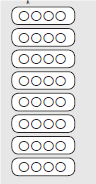Lets the learners work on their array diagrams.

Walks around to see that all learners are working correctly.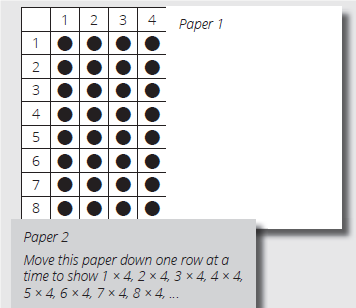Lets the learners move Paper 2 down row by row, one row at a time, counting while

they do this:

1 × 4 = 4, 2 × 4 = 8, 3 × 4 = 12, … , 8 × 4 (4, 8, 12, …, 32).

Shows the movement of Paper 2 on the large array diagram on the board

Lets the learners write the number sentence and answer in their classwork books. (8 × 4 = 32, 32 wheels)

Lets a learner write the number sentence and the answer on the board for correction.

Learners must remember to write the answer with the correct unit.

CLASS ACTIVITY

The teacher

Sayd: Let’s complete our multiplication tables today.

Follow the same steps in the previous lesson to complete the 4 and 5 times table on their multiplication tables.

Ask for random multiples. Learners must use their completed multiplication tables to

a 3 × 5,

b 5 × 4,

c 6 × 3

d 8 × 2..

Pupils pay attention and participate

STEP 3

CLASS-WORK

NOTE: Give each pair of learners a set of multiplication cards (×2, ×3, ×4 and ×5). The cards should have been cut up and prepared in lessons 9, 12, 16 and 18.

Rules of the game

1 Both learners pick up a card with the number sentence side facing up and say the answer to the number sentence on the card.

2 The learner who gets the bigger answer is the winner.

Play the 2 to 5 multiplication card game. Your teacher will explain the rules.

Pupils attempt their class work

STEP 4

HOME-WORK

Complete the following table.

 What is Answer 1 5 x 4 = 2 3 x 5 = 3 5 x 2 = 4 7 x 4 = 5 6 x 3 =

The pupils writes it in their homework book

STEP 5

SUMMARY

The teacher summarizes by reminding the pupils that in today’s lesson we have learnt to solve multiplication problems.

PERIOD 5: Weekly Test/consolidations

TEACHER’S ACTIVITY: The teacher revises all the concepts treated from period 1-4 and gives the pupils follow through exercises, quiz and tests. She marks the exercises, makes corrections and commends the pupils positively.

PUPIL’S ACTIVITY: The pupils work on the worksheets and exercises given by the teacher individually

CONSOLIDATION

NOTE: Learners should use the array diagrams and multiplication table to help them solve

the problems on this activity. It is good if some learners are able to do these calculations

mentally. They can check their answers on the multiplication table.

1 There are 5 groups of children. There are 3 children in each group. How many children

are there altogether?

2 Calculate using the multiplication tables.

a 9 × 2 =

b 8 × 3 =

c 8 × 2 =

d 7 × 4 =

e 7 × 2 =

f 9 × 4 =

g 7 × 5 =

h 7 × 3 =

i 9 × 3 =

j 6 × 4 =